Courses

# Simplification And Approximation - Practice Test

## 5 Questions MCQ Test Quantitative Aptitude for Banking Preparation | Simplification And Approximation - Practice Test

Description
This mock test of Simplification And Approximation - Practice Test for UPSC helps you for every UPSC entrance exam. This contains 5 Multiple Choice Questions for UPSC Simplification And Approximation - Practice Test (mcq) to study with solutions a complete question bank. The solved questions answers in this Simplification And Approximation - Practice Test quiz give you a good mix of easy questions and tough questions. UPSC students definitely take this Simplification And Approximation - Practice Test exercise for a better result in the exam. You can find other Simplification And Approximation - Practice Test extra questions, long questions & short questions for UPSC on EduRev as well by searching above.
QUESTION: 1

### A man has Rs. 480 in the denominations of one-rupee notes, five-rupee notes and ten-rupee notes. The number of notes of each denomination is equal. What is the total number of notes that he has ?

Solution: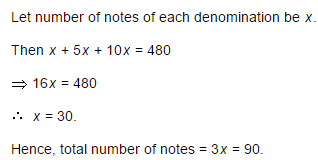QUESTION: 2

### There are two examinations rooms A and B. If 10 students are sent from A to B, then the number of students in each room is the same. If 20 candidates are sent from B to A, then the number of students in A is double the number of students in B. The number of students in room A is:

Solution:

Let the number of students in rooms A and B be x and y respectively.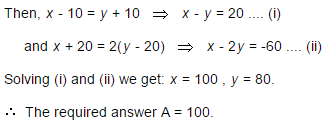QUESTION: 3

### The price of 10 chairs is equal to that of 4 tables. The price of 15 chairs and 2 tables together is Rs. 4000. The total price of 12 chairs and 3 tables is:

Solution:

Let the cost of a chair and that of a table be Rs. x and Rs. y respectively.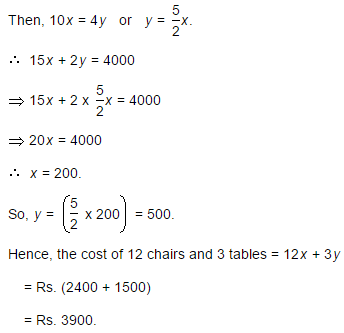QUESTION: 4

If a - b = 3 and a2 + b2 = 29, find the value of ab.

Solution: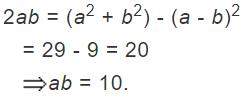QUESTION: 5

The price of 2 sarees and 4 shirts is Rs. 1600. With the same money one can buy 1 saree and 6 shirts. If one wants to buy 12 shirts, how much shall he have to pay ?

Solution:

Let the price of a saree and a shirt be Rs. x and Rs. y respectively.

Then, 2x + 4y = 1600 .... (i)

and x + 6y = 1600 .... (ii)

Divide equation (i) by 2, we get the below equation. => x + 2y = 800. --- (iii)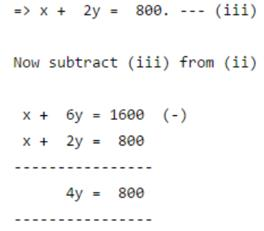Therefore, y = 200. Now apply value of y in (iii) => x + 2 x 200 = 800 => x + 400 = 800 Therefore x = 400

Solving (i) and (ii) we get x = 400, y = 200.

∴ Cost of 12 shirts = Rs. (12 x 200) = Rs. 2400.

Track your progress, build streaks, highlight & save important lessons and more!

### Similar Content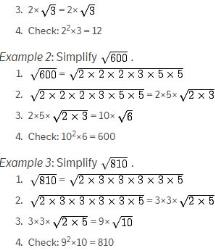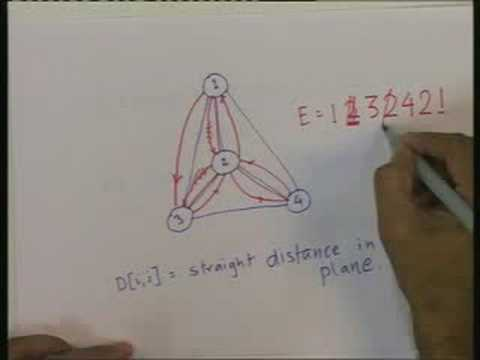### Related tests